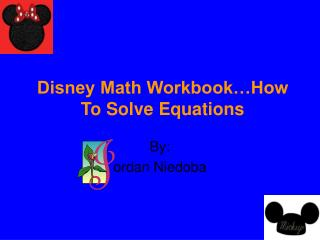# Disney Math Workbook…How To Solve Equations - PowerPoint PPT PresentationDownload PresentationDisney Math Workbook…How To Solve Equations

Disney Math Workbook…How To Solve EquationsDownload Presentation## Disney Math Workbook…How To Solve Equations

- - - - - - - - - - - - - - - - - - - - - - - - - - - E N D - - - - - - - - - - - - - - - - - - - - - - - - - - -
##### Presentation Transcript

1. Disney Math Workbook…How To Solve Equations By: ordan Niedoba

2. How To Solve One-Step Equations • To solve a one-step equation, do the inverse operation to both sides in order to get the variable by itself. • Remember to check your solution • Example:4x=124 4=x 124=32 • Check:4x+124 4 x 32=124 X=124 4 4

3. How To Solve A Two-Step Equations • To solve a two-step equation, first, divide your problem by the equal sign. • Next, circle your variable. • Third, get rid of the number next to the variable by using addition or subtraction. • Finally, multiply or divide. Example: x-7=-9 7+7=x -9+7=-2 x=-2 5 x 5=x -2 x 5=-10 Check: x-7=-9 -10-7=-9 -2-7=-9 x=-10 5 5

4. Solving One-Step Equations • Directions: Mickey Mouse needs help figuring out these equations. Use your best math skills to help him. • 84=x+90 • H-29=26 • -52=r • -24k=144 • C=-60 -11 5

5. One-Step Equation Word Problems • Pooh needs 10 pots of honey to get him through the winter. He already has 3 pots. How many more pots does Pooh need? • Cinderella needs 16 pairs of class slippers. Prince Charming gave her 4 pairs. How many more pairs of class slippers does Cinderella need? • 20 Disney villains were waiting in line to go on Spaceship Earth. 8 of them got on. How many villains still need to ride Spaceship Earth?

6. Solving Two-Step Equations • Directions: Donald Duck is stuck in a wall after a ride that he went on. So now he can’t do his math worksheet for Daisy. Help Donald by solving these equations. • 4x-8 • 4c-12=20 • 20=3x • 10-6v • 8n+7

7. Two-Step Equation Word Problems • 4 friends went to Walt Disney World. While there, they bought tickets and a hotel room. Each person also bought a souvenir for \$25. The total that each person paid was \$1,500. What was the cost for there tickets and hotel room? • Rabbit needs 1,000 carrot seeds to plant a garden. Rabbit already has 600 seeds. 20 seeds come in a packet. How many packets does Rabbit need to buy to have 1,000 seeds? • Minnie, Pluto, and Goofy are baking cakes. They backed 3 cakes. They already had 3 slices from another cake. They need a total of 30 slices of cake. How many slices do they need from each cake?

8. Answer Key • Slide 4: 1. x=6 2. h=55 3. r=572 4. k=-6 5. c=-12 • Slide 5: 1. 7pots 2. 12pairs 3. 12villians • Slide 6: 1. x=8.25 2. c=8 3. x=7.33 4. v=-15.66 5. n=3 • Slide 7: 1. \$6,100 2. 20 packets 3. 9pieces